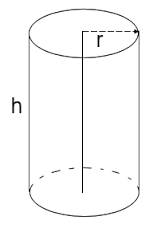Cylinder Calculator

Calculate the various properties of a cylinder for given values.

Volume of Cylinder: [ πr²h ]

 Enter the radius : Enter the height : Volume of Cylinder =

Curved Surface Area (CSA) of Cylinder: [ 2πrh ]

 Enter the radius : Enter the height : Curved Surface Area (CSA) of Cylinder =

Total Surface Area (TSA) of Cylinder: [ 2πr(h+r) ]

 Enter the radius : Enter the height : Total Surface Area (TSA) of Cylinder =

Calculate the various properties of a cylinder like like volume, curved surface area(CSA) and total surface area(TSA) for given values.Formula:

Volume : [ πr²h ]

CSA : [ 2πrh ]

TSA : [ 2πr(h+r) ]

Where

h = height

V = volume

π = pi = 3.1415926535898

CSA = curved surface area

TSA = total surface area

Derivations:

1. Curved Surface Area of Cylinder
In order to find the area of the curved surface area of cylinder we need to know the values of radius and height having same units like mm, cm ,m , ft etc. Cylinder is a 3D structure having circular bases which are parallel to each other. The cylinder can be seen as a set of circular objects that are stacked on one another constitutes the height (h). The circular base is having radius (r) or diameter (d=2r).
Area of the curved surface = Circumference x hight
Therefore CSA = 2πr x h = 2πrh

2. Total Surface Area of Cylinder
As we see in picture cylinder is having one curved surface area and two circular bases (top side and bottom side or call face area).
therefore Total Surface area = Curved Surface area + Area of Circular bases.
Since there are two circular bases, therefore the area of both the circular bases = πr2+πr2 = 2πr2
Now TSA = 2πrh + 2πr2
= 2πr(h + r)